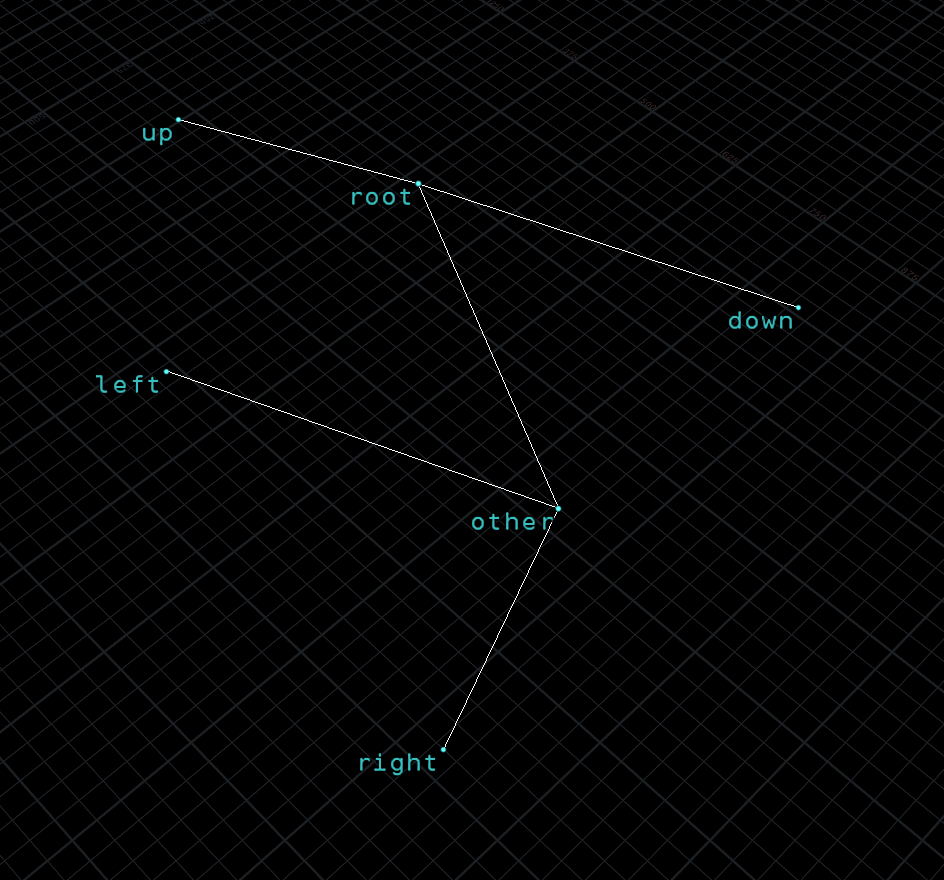reaction diffusion (again)

I took a old solver from about 6 years ago and finally updated it to opencl, so much faster

I didn’t bother to do a exact speed comparison, but its pretty substantial.

I then moved on to doing this in opencl on a 3D grid, not sure the implementation is correct but it generates some interesting results.

It took me a bit to change the 5 point stencil to a 7 point for the volume grid, I originally tried it with connected point grid but things got really weird trying to reuse the 2D versions code which is based on connected points

bootleg twee parser for houdini

I wanted to import a network of twee passages into Houdini to view as a connected graph

I used twee2 the command line tool to decompile twine2 gui html files

I the tw2 was a lot easier to strip apart in python.each tagged passage become a node in the graph, originally each passage became a node, but I wanted some combined.a bigger node tree

Just drop the code in a python sop and point it to the tw2 file, might work?

box packing 2D maps

I wanted a way to remove overlaps from some random rectangles.each box getting pushed out, its a bunch of packed primitives with a vector attribute for width and height

Here is the vex code – run once in a detail wrangle it loops a number of times over the points and pushes them outside while checking for overlaps

int relax_iter=chi("iter");

vector pp[];

for(int i=0;i<npoints(0);i++){
vector p1=point(0,"P",i);
push(pp,p1);
}

for(int q=0;q<relax_iter;q++){
vector newP={0,0,0};
int in=0;
for(int i=0;i<npoints(0);i++){
for(int j=0;j<npoints(0);j++){

vector min1 = pp[i]-point(0,"dimen",i);
vector max1 = pp[i]+point(0,"dimen",i);
vector p1 = pp[i];

float x1min = min1.x;
float x1max = max1.x;
float z1min = min1.z;
float z1max = max1.z;

vector min2 = pp[j]-point(0,"dimen",j);
vector max2 = pp[j]+point(0,"dimen",j);
vector p2 = pp[j];

float x2min = min2.x;
float x2max = max2.x;
float z2min = min2.z;
float z2max = max2.z;

newP = p1+rint(normalize(p1-p2)*4);

in = x1min <= x2max && x2min <= x1max && z1min <= z2max && z2min <= z1max? 1:0;
if(in){
pp[i]=newP;

}
}
}
}

for(int i=0;i<npoints(0);i++){
setpointattrib(0, "P", i, pp[i], "set");
}I also wanted to build a connected structure from this – so I used prim’s algorithm to connect a tree, I disconnected some of the small boxes to keep more primary paths, since the small islands gets connected any way when everything is converted to right angles